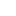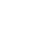# Standardization of NaOH against 0.15N

Standardization of NaOH against 0.15N oxalic acid by Acid Base titration

#### Introduction:

It is a quantitative method of analysis for determining the unknown concentration of an acid or base by exactly neutralizing it with a standard solution of base or acid of known concentration is called an acid-base titration.

#### Theory:

There are the following types of acid-base titration

• Strong acid-base titration
• Strong acid and weak base titration
• Weak acid strong base titration
• Weak acid weak base titration

#### Strong acid-base titration:

Strong acid strong base titration is a quantitative technique used to determine the concentration of a known acidic solution by titrating it with a strongly basic solution of unknown solution concentration and vice versa until neutralization occurs. So there is a strong relationship between a strong acid and a strong base which results in water and salt formation.

#### Strong acid weak base titration:

In strong acid and weak base titration, acid and base will react to form a product that will be produced during this titration. Which then will react to produced a hydronium ion

#### Weak acid strong base titration:

The titration of a weak acid with a strong base involves the direct transfer of a proton from the weak acid to the hydroxide and in this type of titration acid and base react with one of reaction Weak acid weak base

#### titration:

The equivalent point is predicted to be the steepest of the curve as seen in weak acid strong base or acid weak base titration

#### Indicator:

The substance which shows the completion of the reaction is known as an indicator. Phenolphthalein is used as an indicator in most acid-base titrations. It shows the colour change with changing PH. It is colourless which is associated to form pink colour ions.

#### Equivalence point:

The point at which neutralization occur is called the equivalence point. This is the point where the concentration of hydronium ions and hydroxyl ions becomes equal to each other.

#### Endpoint:

The point at which the indicator shows the colour change is called an endpoint.

#### Procedure:

• The apparatus taken was cleaned and washed and fixed accurately
• The burette was filled up to the mark with a standard solution of 0.15 N oxalic acid
• 10 ml of sample solution NaOH was taken in a flask and added few drops of phenolphthalein
• Then sodium hydroxide was titrated against the oxalic acid volume of acid used in endpoint was noted
• Repeated the experiment three times to take a mean and accurate reading. Then by using the formula N1V1 = N2V2 unknown normality can be calculated
• normality of sodium hydroxide was calculated

#### Observation and Calculations:

Preparation of 0.15 N solution of oxalic acid

(COOH)2 (COO) ₊ 2H⁺

So there are two replaceable ions in oxalic acid

The equivalent weight of oxalic acid = 126.02/2

= 63.035 g

1000 ml of 1N solution contains oxalic acid= 63.05 g

1 ml……………….. = 63.05/1000

100ml ……… = 63.05 × 100/1000

= 6.30 g

So 6.30 g of oxalic acid was weighed and dissolved in a measuring flask and fill it up to the mark.

Standardization of NaOH against 0.15N oxalic acid by Acid-Base titration

#### Chemical equation:

(COOH)2 + 2NaOH → (COONa)2 + 2H2O

The volume of NaOH used = 10 ml

 Sr. No Initial reading (ml) Final reading (ml) Volume used 1 0 9.9 9.9 2 9.9 20 10.1 3 20 30.6 10.6

Mean volume = 9.9 + 10.1 +10.6/ 3

= 10.2 ml

Acid = Base

N1V1 = N2V2

0.15 × 10.2 = N2 × 10

N2 = 0.153 N

#### Systematic error and precaution:

During the performance of experiment, a number of systematic and individual error may occur such as systematic constant and random errors these errors disturb and alter the result of experiment determinate errors can be minimised by using different techniques and also by extensive care and by finding fault in their concentration and buy calibration and use of proper solvent

#### Results:

The normality of NaOH is 0.153N

Standardization of NaOH against 0.15N oxalic acid by Acid-Base titration###### Don’t miss these Chemistry topics!
Sharing Is Caring:

### 5 thoughts on “Standardization of NaOH against 0.15N”

1.So helpful content i was looking for this this help me very much

•Thanks

2.whoah this blog is wonderful i love reading your articles. Keep up the great work! You know, many people are looking around for this information, you can aid them greatly.

3.•